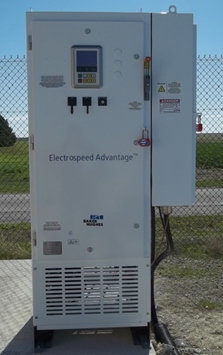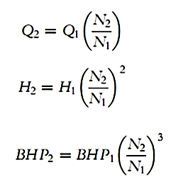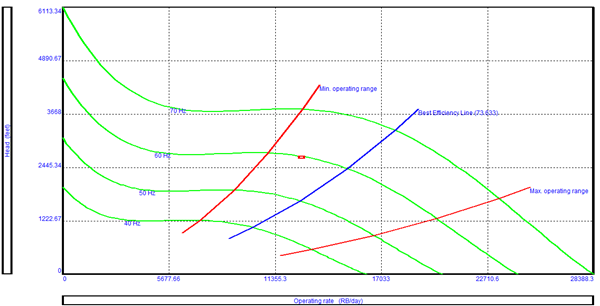ESP design – Step 9: Variable Speed Submersible Pumping SystemCompared to conventional ESP installations with constant motor speeds, installations running at variable frequencies have several advantages. The most important benefit of a Variable Speed Submersible Pumping System is the wide flexibility of the variable frequency ESP system that permits perfect matching of the lift capacity of the ESP system and the well’s productivity. Therefore, it operates over a much broader range of capacity, head, and efficiency.NB: Variable Frequency Drive basics (also, named: Variable Speed Drive) are presented and discussed in the article “Variable Frequency Drive Basics”.

Since a submersible pump motor is an induction motor, its speed is proportional to the frequency of the electrical power supply. This relationship between variables involved in pump performance (such as head, flow rate, shaft speed) and power is known as “Affinity Laws” (also called “Pump Laws”).

According to the affinity laws, the following relationships exist between the actual speed of the centrifugal pump and its most important performance parameters:

• The flow rate of a pump changes directly proportional to its operating speed.
• The head developed by the pump changes proportionally to the square of the speed.
• The brake horsepower required to drive the pump changes proportionally to the cube of the speed.
• The efficiency of the pump does not change with speed changes.

The above rules can be expressed by the following equations:• N1 and N2 are pumping speeds (in RPM).
• Q1 and Q2 are pumping rates at pumping speeds N1 and N2 (in bpd).
• H1 and H2 are the developed heads at pumping speeds N1 and N2 (in ft).
• BHP1 and BHP2 are the required brake horsepower at pumping speeds N1 and N2 (in hp).

How can Affinity Laws be used to design the pump?

Variable Speed Drives generate frequency goes from 30 to 90 Hz. Changing the operating frequency will change the motor speed, the pumping speed will be changed, so that the developed head and the required brake horsepower will change. Thus pump performance curves for frequencies other than 60 Hz (or 50 Hz) can be generated.

Each curve represents a series of points derived from the 60 Hz curve for flow and corresponding head points, transformed using the Affinity Law Equations.